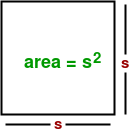Open in App
Not now

# Program to find the area of a Square

• Difficulty Level : Easy
• Last Updated : 21 Jun, 2022

What is a square ?
Square is a flat shape, in one plane, defined by four points at the four corners. A square has four sides all of equal length, and four corners, all right angles (90 degree angles). A square is a kind of rectangle but with all sides equal.Formula :Examples:

```Input : 4
Output :16

Input :8
Output :64```

## CPP

 `// CPP program to find the area of a square``#include ``using` `namespace` `std;` `// function to find the area``int` `area_fun(``int` `side)``{``int` `area = side * side;``return` `area;``}` `// Driver program``int` `main()``{``int` `side = 4;``int` `area = area_fun(side);``cout << area;``return` `0;``}`

## Java

 `// Java program to find``// the area of a square` `class` `GFG``{` `// function to find the area``static` `int` `area_fun(``int` `side)``{``int` `area = side * side;``return` `area;``}` `// Driver code``public` `static` `void` `main(String arg[])``{``    ``int` `side = ``4``;``    ``int` `area = area_fun(side);``    ``System.out.println(area);``}``}` `// This code is contributed``// by Anant Agarwal.`

## Python3

 `# Python program to find``# the area of a square` `# function to find the area``def` `area_fun(side):``    ``area ``=` `side ``*` `side``    ``return` `area` `# Driver program``side ``=` `4``area ``=` `area_fun(side)``print` `(area)` `# This Code is Contributed``# by Azkia Anam.`

## C#

 `// C# program to find``// the area of a square``using` `System;` `class` `GFG {` `    ``// function to find the area``    ``static` `int` `area_fun(``int` `side)``    ``{``        ``int` `area = side * side;``        ``return` `area;``    ``}` `    ``// Driver code``    ``public` `static` `void` `Main()``    ``{``        ``int` `side = 4;``        ``int` `area = area_fun(side);``        ``Console.WriteLine(area);``    ``}``}` `// This code is contributed by vt_m.`

## PHP

 ``

## Javascript

 ``

Output:

`16`

Time complexity: O(1)

space complexity: O(1)
This article is contributed by Ajay Puri. If you like GeeksforGeeks and would like to contribute, you can also write an article using write.geeksforgeeks.org or mail your article to review-team@geeksforgeeks.org. See your article appearing on the GeeksforGeeks main page and help other Geeks.